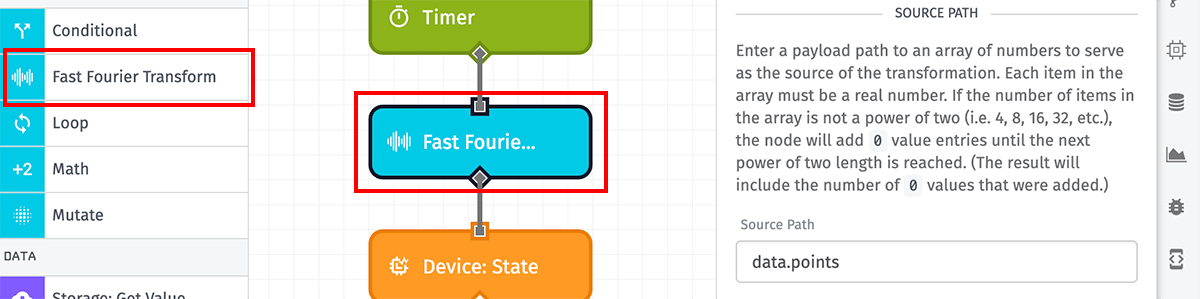# Fast Fourier Transform Node

The Fast Fourier Transform Node allows for transforming an array of data points from one domain (such as space or time) into a frequency representation using a fast Fourier transform algorithm.## Configuration

The Fast Fourier Transform Node takes three properties for its configuration.

### Source Path

First, enter a payload path to an array of numbers on which to run the transformation. Each item in the array must be a real number, or the operation will fail.

Ideally, the length of the array should be a power of 2 — as in, the transformation should be applied to 2, 4, 8, 16, 32, 64, 128, etc. numbers. If the array length is not a power of 2, the node will add `0` values to the array until the next power of 2 length is reached.

Note: The maximum length of the input array is 2,048 items. Attempting to transform an array of more items will cause the node to error.

### Decimal Place Rounding

Next, you may optionally select between the ten millionths (7th decimal digit) and ones (whole number) to round the output values to. By default no rounding will occur, and the values will be double-precision floating-point numbers.

### Result Path

Next, enter a payload path where the result of the transformation should be placed. The result will be an object containing the following properties:

• result: An array containing the calculated frequency domains.
• sampleCount: The length of the provided array of numbers.
• paddingCount: The amount of zeroes added to a non-power-of-two input array length.

## Node Errors

There are a handful of common cases where the Fast Fourier Transform Node will throw an error:

• If the value at the source path is not an array.
• If the value is an array but the array is empty.
• If any item within the source array is not a number.
• If the length of the source array is greater than 2,048 items.